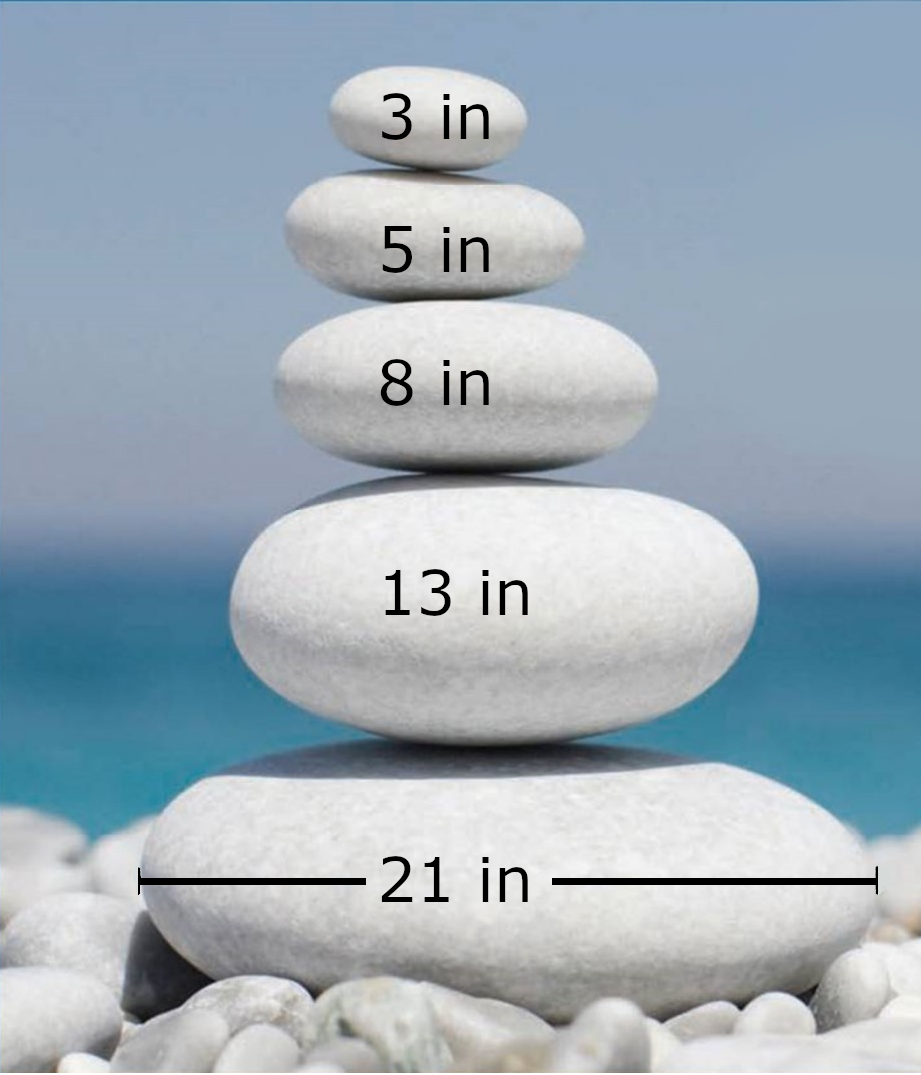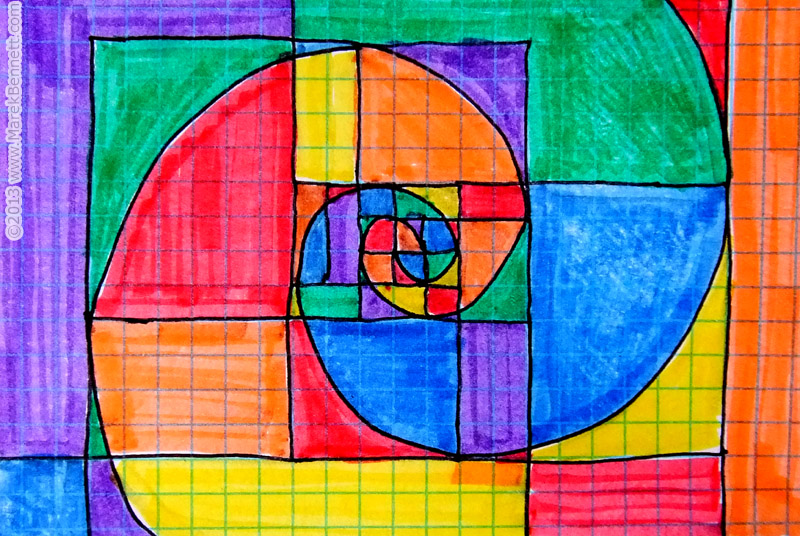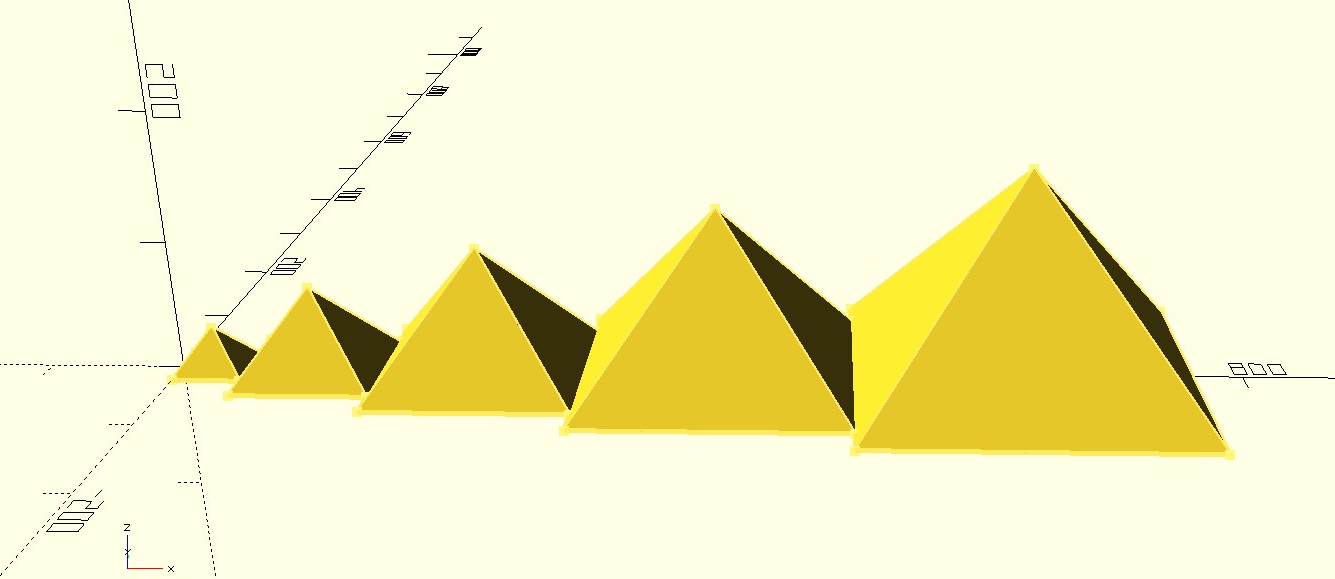## Fibonacci ArtSource: Karin Henseler from Pixabay

Type: Investigation

Theme: Art

Grades: 5, 6, 7, 8, 9, 10

Learning Target: Students can recognize recursive sequences and creatively apply Fibonacci squares or the Golden Mean in an art project.Instructions

For this investigation each student will create one art project (line, color, mixed media, 3D, or computer-generated).

1. Students will make a list of recursive values they use in their artwork. They must include at least 5 iterations.

2. Students will use these values to construct shapes, objects, or design elements using a centimeter ruler or grid paper.

3. Students will submit their artwork as a photograph, scan or digital file.

4. Explanation

1. Students will write at least one paragraph (minimum 3 sentences) explaining how the Fibonacci Sequence or the Golden Mean applies to their artwork.

2. Students will include their list of recursive values.

### Rubric

• Completeness (Were the requirements above fulfilled?)

• Clarity (Is the artwork neat, organized, and interesting to look at?)

• Concepts (Has the student explained how the Fibonacci sequence or the Golden Mean applies to their artwork?)

• Accuracy (Are there recursive sequence values correct?)

## Examples and Ideas

### Fibonacci Sequence

1. One way to set up your project is to make a list of recursive values based on the Fibonacci sequence, 1 in, 2 in, 3 in, 5 in, 8 in, 13 in. Then construct your artwork based on those values.

2. Similarly you could draw Fibonacci squares on paper, color them, cut them out, and rearrange them on paper in an interesting design.

### The Golden Mean

You may also use the Golden Mean (Ratio) to determine your sizes. Remember the ratio between each successive size will be phi (Φ, the Golden Mean, which is 1.618...).

1. Select the smallest size of your object’s dimension, such as 2 cm.

2. Next multiple each dimension recursively by Φ (round to 1.6).

For 2 cm, the results would be:

2 cm

2 cm x 1.6 =   3.2 cm

3.2 cm x 1.6 =   5.1 cm

5.1 cm x 1.6 =   8.2 cm

8.2 cm x 1.6 = 13.1 cm

13.1 cm x 1.6 = 21.0 cm

Rocks of the proper dimensions (width) are stacked to produce an interesting design (top).

Fibonacci squares and spirals are colored and organized in an interesting design (middle).

```initial = 10;
phi = 1.618;
dia= PI*initial/2;
base = sqrt(2*pow(dia,2));
height = 2*initial;
for (i=[1:5]){
scale([i*phi,i*phi,i*phi])
nbsp;       translate([i*dia,0,0])
rotate([0,0,45])<
cylinder(height,base,00,\$fn=4);
}```Exit Ticket
CCSS Math Practice
• I can look for and express regularity in repeated reasoning.
NGSS Crosscutting Concepts
• Patterns

### Samples

Samara's Fibonacci Project
Henrielle's Fibonacci Project